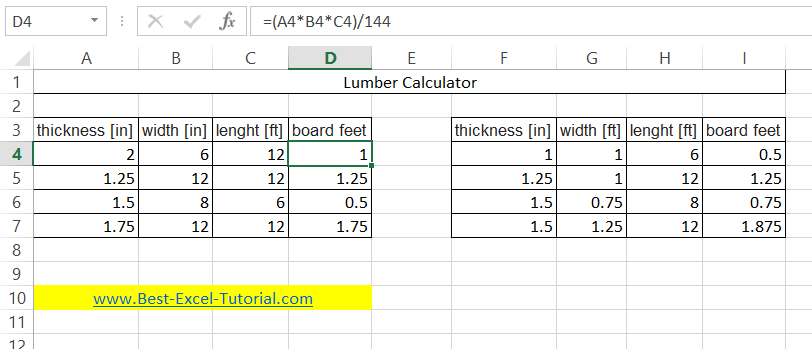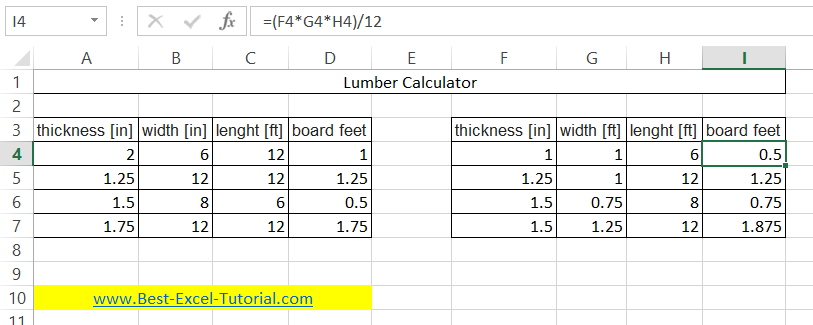#### Board Footage Calculator

In this Excel lesson you will teach yourself how to prepare Board Footage Calculator.

## What is board footage?

Board footage is a volume of hardwood lumber (to buy).

One board foot equals one square foot of wood which is one inch thick. Here is the relationship:

1 board foot = 144 cubic inches = 1/12 cubic foot

To prepare such lumber calculations you need to prepare the data first. You will need:

• board length [feet]
• width [ft or inches]
• thickness [in]
`If you prefer metric units you may need cm to inches converter.`

## How to calculate board footage?

To calculate wood board footage you just need to multiply length * width * thickness.

Board foot formula = length * width * thickness

To adjust units you need to use these formulas:

board foot = length(ft) * width(in) * thickness(in) / 144board foot = length(ft) * width(ft) * thickness(in) / 12To format the board footage as a number with 2 decimal places, select the cell, right-click, and select “Format Cells”. In the Format Cells dialog box, select the “Number” category, choose the number format with 2 decimal places, and click OK.

Now you know how to calculate board feet.

## Expand for Multiple Boards

Expanding your Excel spreadsheet to calculate board footage for multiple boards is a useful way to streamline the process, especially when dealing with multiple pieces of lumber in a project. Here’s how you can expand your table to calculate board footage for multiple boards:

### Step 1: Add More Rows

Below the row where you entered data for the first board, add additional rows for each additional board. You can do this by right-clicking on the row number and selecting “Insert” or simply by clicking on the row number and pressing “Ctrl” + “+” (Windows) or “Cmd” + “+” (Mac) on your keyboard.

### Step 2: Enter Data for Each Board

In the newly added rows, enter the measurements for each board in the appropriate columns. For example, if you have data for three boards, you would enter the measurements in rows 2, 3, and 4.

### Step 3: Calculate Board Footage for Each Board

Now that you have data for multiple boards, you can apply the same board footage formula to each row to calculate board footage for each board individually. Assuming your data for the second board is in cells B3, C3, and D3 and for the third board in cells B4, C4, and D4, you can use the following formulas:

For the second board (cell E3):

= (B3 * C3 * D3) / 144

For the third board (cell E4):

= (B4 * C4 * D4) / 144

These formulas will calculate board footage for each board based on their respective dimensions.

### Step 4: Format the Board Footage

Follow the same formatting steps mentioned earlier to format the calculated board footage cells with two decimal places.

By following these steps, you can efficiently calculate board footage for multiple boards within your Excel spreadsheet. This method allows you to quickly estimate the total volume of lumber required for your project, making it a valuable tool for woodworking and construction tasks.